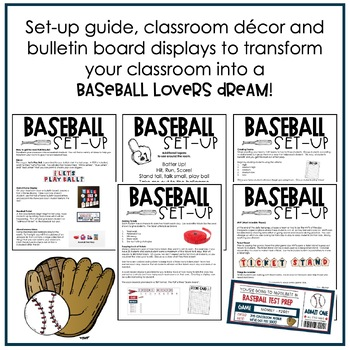# 5th Grade Math Test Prep Activities | Baseball Test Prep Room Transformation5th
Subjects
Standards
Resource Type
Formats Included
• Zip
Pages
175+

### Description

Make 4th-grade math test prep fun with a test prep baseball theme!

Why the baseball math test prep?

Students will be highly engaged and won’t even realize that they are participating in test prep! Within the resource, I provide a step-by-step approach that I follow, but you may find a way that suits you better!

Every 4th-grade math standard* is addressed. Students answer multiple-choice and short answer math questions to earn tickets for teamwork, perseverance, efficiency, showing work, and using test-taking strategies. Then tickets can be used during game day!

What’s included in the 4th Grade Math Baseball Test Prep?

58-pages of test-like questions (4 questions per standard!)

Implementation Guide

Printable classroom décor

Let’s Play Ball Banner

Hall of Fame Display

Score Board Bulletin Board Display

Ticket Stand Banner

Bulletin Board Letters in Red, White, and Blue

Eight low prep games

Step-by-step directions

Game pieces

Test-taking strategies poster

Ticket poster

Recording sheets

Motivational wrist bands

Test day notes

Testing signs

Do you have your own test prep materials and only want to transform your room? Find the Baseball Test Prep Room Transformation Kit HERE!

ALSO INCLUDED:

If you are looking for the original Amazing Race Test Prep resource and all its components, it’s included in the download!

*Since most state standards are similar to the Common Core Math Standards, the math test prep questions are aligned to Common Core.

Customer Tips: Look for the green star next to my logo and click it to become a follower and receive email updates!

Still have questions? Email me at mandy@teachingwithsimplicity.com, and I would love to help you out!

Total Pages
175+
Included
Teaching Duration
Lifelong tool
Report this Resource to TpT
Reported resources will be reviewed by our team. Report this resource to let us know if this resource violates TpT’s content guidelines.

### Standards

to see state-specific standards (only available in the US).
Use parentheses, brackets, or braces in numerical expressions, and evaluate expressions with these symbols.
Write simple expressions that record calculations with numbers, and interpret numerical expressions without evaluating them. For example, express the calculation “add 8 and 7, then multiply by 2” as 2 × (8 + 7). Recognize that 3 × (18932 + 921) is three times as large as 18932 + 921, without having to calculate the indicated sum or product.
Generate two numerical patterns using two given rules. Identify apparent relationships between corresponding terms. Form ordered pairs consisting of corresponding terms from the two patterns, and graph the ordered pairs on a coordinate plane. For example, given the rule “Add 3” and the starting number 0, and given the rule “Add 6” and the starting number 0, generate terms in the resulting sequences, and observe that the terms in one sequence are twice the corresponding terms in the other sequence. Explain informally why this is so.
Add and subtract fractions with unlike denominators (including mixed numbers) by replacing given fractions with equivalent fractions in such a way as to produce an equivalent sum or difference of fractions with like denominators. For example, 2/3 + 5/4 = 8/12 + 15/12 = 23/12. (In general, 𝘢/𝘣 + 𝘤/𝘥 = (𝘢𝘥 + 𝘣𝘤)/𝘣𝘥.)
Solve word problems involving addition and subtraction of fractions referring to the same whole, including cases of unlike denominators, e.g., by using visual fraction models or equations to represent the problem. Use benchmark fractions and number sense of fractions to estimate mentally and assess the reasonableness of answers. For example, recognize an incorrect result 2/5 + 1/2 = 3/7, by observing that 3/7 < 1/2.